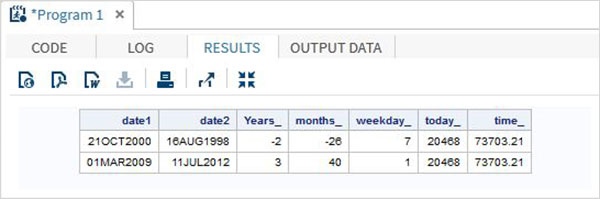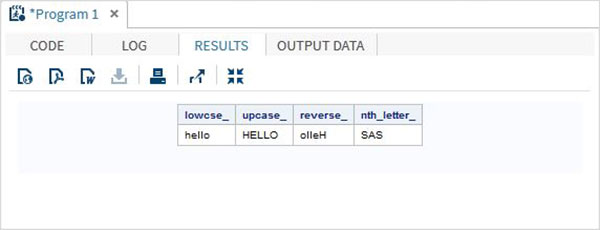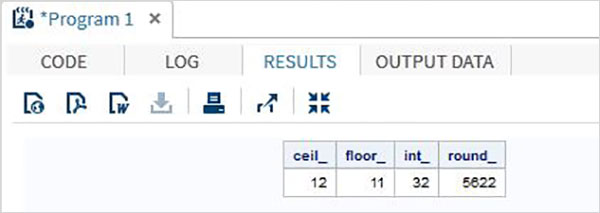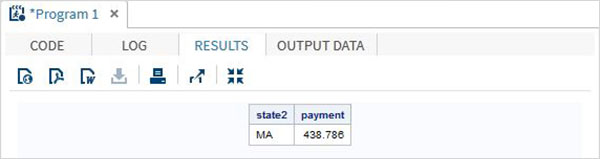# SAS - Functions

SAS has a wide variety of in built functions which help in analysing and processing the data. These functions are used as part of the DATA statements. They take the data variables as arguments and return the result which is stored into another variable. Depending on the type of function, the number of arguments it takes can vary. Some functions accept zero arguments while some other accept fixed number of variables. Below is a list of types of functions SAS provides.

### Syntax

The general syntax for using a function in SAS is as below.

```FUNCTIONNAME(argument1, argument2...argumentn)
```

Here the argument can be a constant, variable, expression or another function.

## Function Categories

Depending on their usage, the functions in SAS are categorised as below.

• Mathematical
• Date and Time
• Character
• Truncation
• Miscellaneous

## Mathematical Functions

These are the functions used to apply some mathematical calculations on the variable values.

### Examples

The below SAS program shows the use of some important mathematical functions.

data Math_functions;

```v1=21; v2=42; v3=13; v4=10; v5=29;

/* Get Maximum value */
max_val = MAX(v1,v2,v3,v4,v5);

/* Get Minimum value */
min_val = MIN (v1,v2,v3,v4,v5);

/* Get Median value */
med_val = MEDIAN (v1,v2,v3,v4,v5);

/* Get a random number */
rand_val = RANUNI(0);

/* Get Square root of sum of the values */
SR_val= SQRT(sum(v1,v2,v3,v4,v5));

proc print data = Math_functions noobs;
run;
```

When the above code is run, we get the following output −## Date and Time Functions

These are the functions used to process date and time values.

### Examples

The below SAS program shows the use of date and time functions.

```data date_functions;
INPUT @1 date1 date9. @11 date2 date9.;
format date1 date9.  date2 date9.;

/* Get the interval between the dates in years*/
Years_ = INTCK('YEAR',date1,date2);

/* Get the interval between the dates in months*/
months_ = INTCK('MONTH',date1,date2);

/* Get the week day from the date*/
weekday_ =  WEEKDAY(date1);

/* Get Today's date in SAS date format */
today_ = TODAY();

/* Get current time in SAS time format */
time_ = time();
DATALINES;
21OCT2000 16AUG1998
01MAR2009 11JUL2012
;
proc print data = date_functions noobs;
run;
```

When the above code is run, we get the following output −## Character Functions

These are the functions used to process character or text values.

### Examples

The below SAS program shows the use of character functions.

```data character_functions;

/* Convert the string into lower case */
lowcse_ = LOWCASE('HELLO');

/* Convert the string into upper case */
upcase_ = UPCASE('hello');

/* Reverse the string */
reverse_ = REVERSE('Hello');

/* Return the nth word */
nth_letter_ = SCAN('Learn SAS Now',2);
run;

proc print data = character_functions noobs;
run;
```

When the above code is run, we get the following output −## Truncation Functions

These are the functions used to truncate numeric values.

### Examples

The below SAS program shows the use of truncation functions.

```data trunc_functions;

/* Nearest greatest integer */
ceil_ = CEIL(11.85);

/* Nearest greatest integer */
floor_ = FLOOR(11.85);

/* Integer portion of a number */
int_ = INT(32.41);

/* Round off to nearest value */
round_ = ROUND(5621.78);
run;

proc print data = trunc_functions noobs;
run;
```

When the above code is run, we get the following output −## Miscellaneous Functions

Let us now understand the miscellaneous functions of SAS with some examples.

### Examples

The below SAS program shows the use of Miscellaneous functions.

```data misc_functions;

/* Nearest greatest integer */
state2=zipstate('01040');

/* Amortization calculation */
payment = mort(50000, . , .10/12,30*12);

proc print data = misc_functions noobs;
run;
```

When the above code is run, we get the following output −